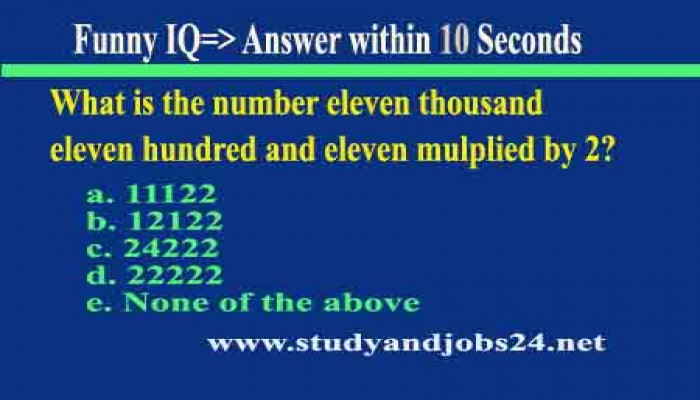What is the number eleven thousand eleven hundred and eleven mulplied by 2?

a. 11122

b. 12122

c. 24222

d. 22222

e. None of the above

The correct answer is c. 24222. Why?

11000* 2 = 22000

1100 *2  =  2200 and

11 *2 =       22

--------------------------

Total      = 24222